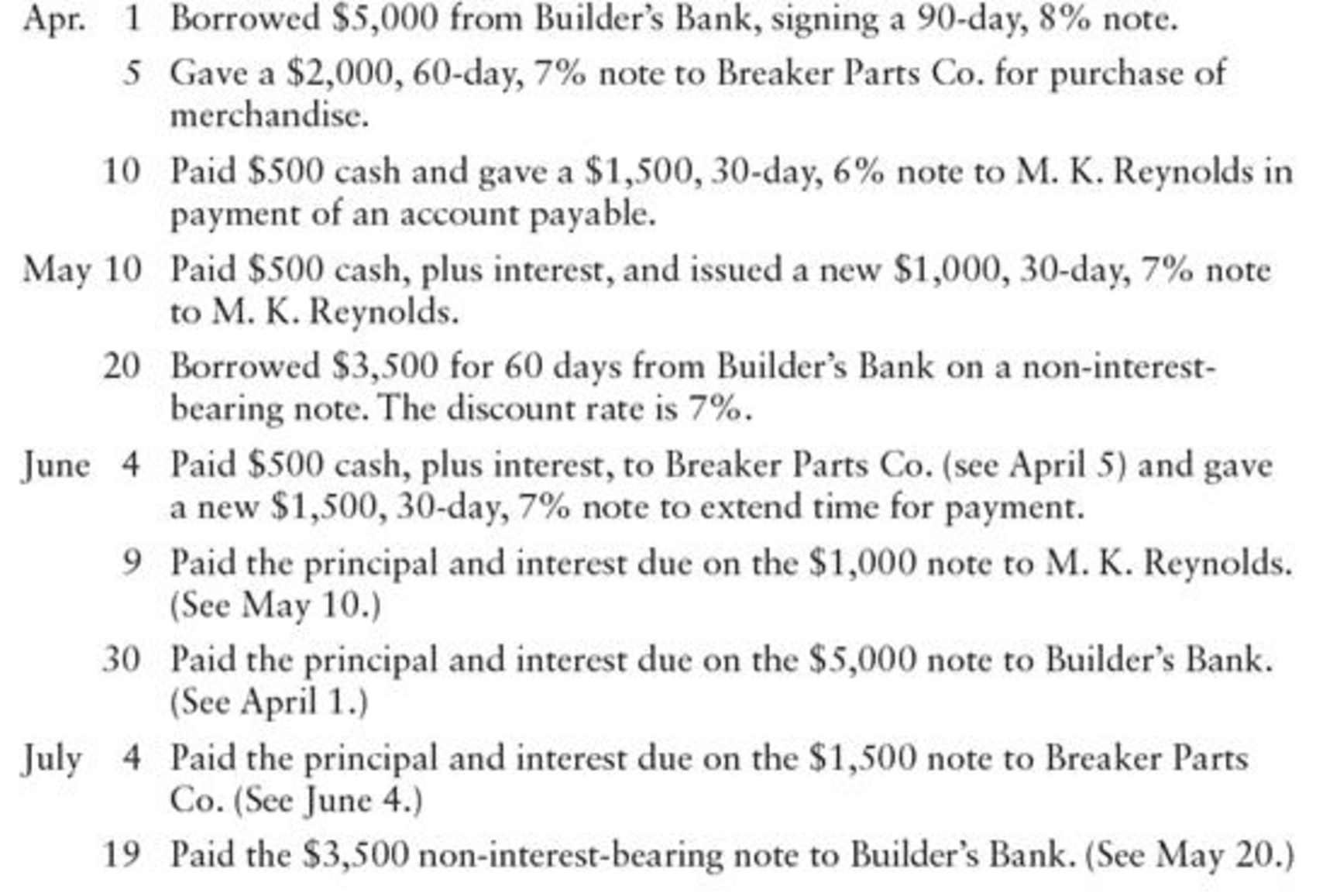Chapter 17, Problem 13SPA

Chapter
Section
Textbook Problem

NOTES PAYABLE ENTRIES Milo Radio Shop had the following notes payable transactions:REQUIREDRecord the transactions in a general journal.

To determine

Prepare journal entry to record the following transactions of MR Shop.

Explanation

Note Payable:

Note payable is an obligation of the business to pay to its creditors in future for the benefits received that carries some interest.

Prepare journal entry to record the following transactions of MR Shop are shown below:

 Date Account titles and Explanation Debit Credit April 1 Cash $5,000 Notes payable$5,000 (To record note issued for bank loan) April 5 Purchases $2,000 Notes payable$2,000 (To record note issued for inventory purchase) April 10 Accounts payable - M.K.R $2,000 Cash$500 Notes payable $1,500 (To record partial payment made and issued note to settle account) May 10 Notes payable (old note)$1,500 Interest expense (1) $7.50 Cash$507.50 Notes payable (new note) $1,000 (To record paid interest and part of partial of principal on old note and issued new note) May 20 Cash (3)$3,459.17 Discount on bonds payable (2) $40.83 Notes payable$3,500 (To record issued note for bank loan) June 4 Notes payable (old note) $2,000 Interest expense (4)$23.33 Cash $523.33 Notes payable (new note)$1,500 (To record paid interest and part of partial of principal on old note and issued new note) June 9 Notes payable $1,000 Interest expense (5)$5.83 Cash $1,005.83 (To record paid note with interest at maturity) June 30 Notes payable$5,000 Interest expense (6) $100 Cash$5,100 (To record paid note with interest at maturity) July 4 Notes payable $1,500 Interest expense (7)$8.75 Cash $1,508.75 (To record paid note with interest at maturity) July 19 Notes payable$3,500 Interest expense (8) $40.83 Discount on notes payable$40.83 Cash $3,500 (To record paid note at maturity) Table (1) Working notes: (1)Calculate interest expenses. Interest expense =Notes payable ×Interest rate×Time period=$1,500×6%×30360=$7.50 (2)Calculate discount on notes payable. Discount on notes payable =Notes payable×Interest rate×Time period=$3,500×7%×60360=\$40

Still sussing out bartleby?

Check out a sample textbook solution.

See a sample solution

The Solution to Your Study Problems

Bartleby provides explanations to thousands of textbook problems written by our experts, many with advanced degrees!

Get Started

What is goal congruence?

Accounting Information Systems

When does a country become an exporter of a good? An importer?

Principles of Macroeconomics (MindTap Course List)

Which fluctuate morelong-term or short-term interest rates? Why?

Fundamentals of Financial Management, Concise Edition (with Thomson ONE - Business School Edition, 1 term (6 months) Printed Access Card) (MindTap Course List)